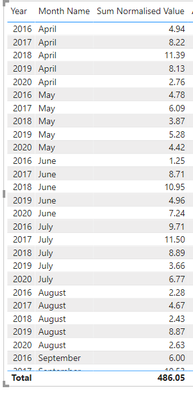cancel
Showing results for
Did you mean:Post Prodigy

## Cumulative sum of average measure

Hi there,

I have got six years of data. Its regulatory year starts on 1st April and finishes on 31st March. I want to calculate the cumulative average of the sum on a monthly basis for the last 5 years (don't want to include the current year). I am doing the following steps to calculate:

Calculate the sum of value using following measure:

Sum Normalised Value = CALCULATE(sum('Fact table'[30 Mins Normalised Value]), FILTER('Date','Date'[Date] <[Current_RY_Yr_Strt] && 'Date'[Date] >= [last 5 years]))
Average of sum using following measure:
Average Normalized Value = AVERAGEX( VALUES('Date'[RY Month Year Sort]),[Sum Normalised Value])
Cumulative sum of average using following measure:

Cumulative Norm Value =
Var current_date = MAX('Fact table'[Date] )
RETURN
SUMX(
FILTER(ALL('Date'),'Date'[Date] <= current_date
&& 'Date'[Date] < [Current_RY_Yr_Strt] ), [Average Normalized Value])

When I ran the report it produces the following output:As you can see that cumulative Norm Value does not cumulative add Average Normalised Value. I am not sure where I made the mistake. Could anyone help me in fixing the issue?

1 ACCEPTED SOLUTIONCommunity Support

Hi @Dunner2020,

According to your description, It sounds like you want to apply multiple aggregates on measure calculation, right?

IF that is the case, I'd like to suggest you add a variable with summarize function to apply the first aggregation, then you can use iterator functions to invoke the above variable table to calculate the second aggregate.

Measure Totals, The Final Word

``````measure =
VAR summary =
SUMMARIZE ( Table, [category 1], [category 2], "AVG", AVERAGE ( Table[Amount] ) )
RETURN
SUMX ( summary, [AVG] )``````

Regards,

Xiaoxin Sheng

Community Support Team _ Xiaoxin
If this post helps, please consider accept as solution to help other members find it more quickly.
2 REPLIES 2Community Support

Hi @Dunner2020,

According to your description, It sounds like you want to apply multiple aggregates on measure calculation, right?

IF that is the case, I'd like to suggest you add a variable with summarize function to apply the first aggregation, then you can use iterator functions to invoke the above variable table to calculate the second aggregate.

Measure Totals, The Final Word

``````measure =
VAR summary =
SUMMARIZE ( Table, [category 1], [category 2], "AVG", AVERAGE ( Table[Amount] ) )
RETURN
SUMX ( summary, [AVG] )``````

Regards,

Xiaoxin Sheng

Community Support Team _ Xiaoxin
If this post helps, please consider accept as solution to help other members find it more quickly.Super User

"I want to calculate the cumulative average"

That's not a thing.  Did you mean to say:  "I want to calculate the average of the monthly sums" ?

Your AVERAGEX measure is missing the monthly bucketing.

Which average are you trying to compute?  Across all months regardless of year?What would be the expected result?